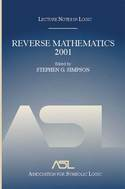# Lecture Notes in Logic, 21

## Reverse Mathematics 2001

Stephen G. Simpson , editorYear: 2005
ISBN: 1-56881-264-7
416 pages. Paperback.

Year: 2005
ISBN:1-56881-263-9
416 pages. Hardcover.

Reverse Mathematics is a program of research in the foundations of mathematics, motivated by the foundational questions of what are appropriate axioms for mathematics, and what are the logical strengths of particular axioms and particular theorems. The book contains 24 original papers by leading researchers. These articles exhibit the exciting recent developments in reverse mathematics and subsystems of second order arithmetic.

• Preface
• Andrew Arana
Possible m-diagrams of models of arithmetic
Weak theories of nonstandard arithmetic and analysis
• Douglas K. Brown
Notions of compactness in weak subsystems of secord order arithmetic
• Douglas Cenzer and Jeffrey B. Remmel
Proof-theoretic strength of the stable marriage theorem and other problems
• Peter A. Cholak, Mariagnese Giusto, Jeffry L. Hirst, and Carl G. Jockusch, Jr.
Free sets and reverse mathematics
• C.T. Chong, Richard A. Shore, and Yue Yang
Interepreting arithmetic in the r.e. degrees under \$\Signma_4\$-induction
• Rodney G. Downey and Reed Solomon
Reverse mathematics, Archimedean classes, and Hahn’s Theorem
• António M. Fernandes
The Baire category theorem over a feasible base theory
• Harvy M. Friedman
Maximal nonfinitely generated subalgebras
• Harvey M. Friedman
Metamathematics of comparability
• Jeffry L. Hirst
A note on compactness of countable sets
• Jeffry L. Hirst
A survey of the reverse mathematics of ordinal arithmetic
• Jeffry L. Hirst
Reverse mathematics and ordinal suprema
• A. James Humphreys
Did Cantor need set theory?
• Julia F. Knight
Models of arithmetic: quantifiers and complexity
• Ulrich Kohlenbach
Higher order reverse mathematics
• Roman Kossak
Arithmetic saturation
• Alberto Marcone
WQO and BQO theory in subsystems of second order arithmetic
• James H. Schmerl
Reverse mathematics and graph coloring:eliminating diagonalization
• James H. Schmerl
Undecidable theories and reverse mathematics
• Stephen G. Simpson
\$\Pi^0_1\$ sets and models of \$WKL_0\$
• Kazuyuki Tanaka and Takeshi Yamazaki
Manipulating the reals in \$RCA_0\$
• Takeshi Yamazaki
Reverse mathematics and wek systems of 0-1 strings for feasible analysis